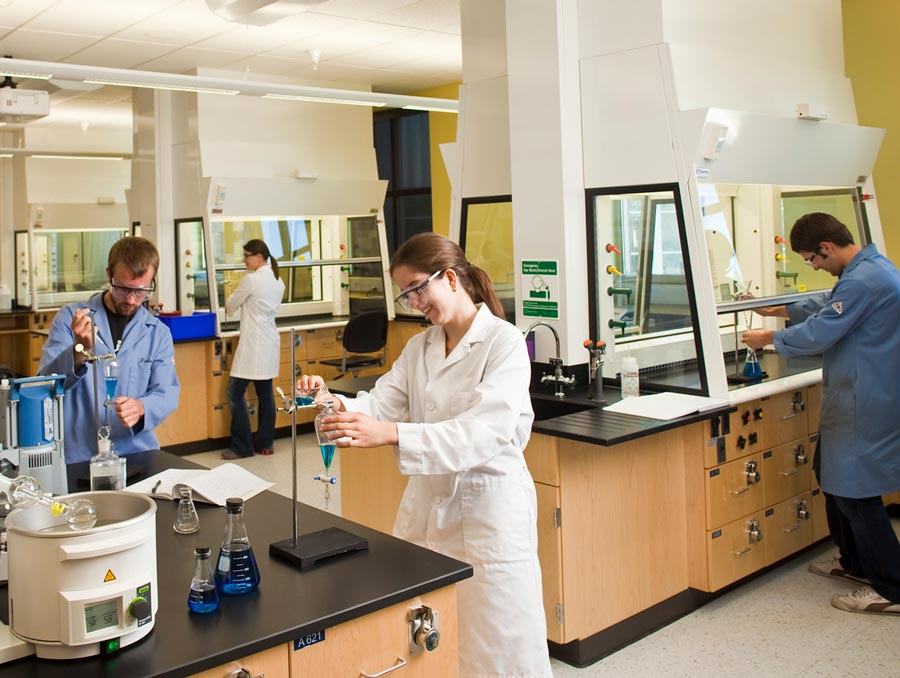# December Science Question of the Month Winner: Daniel Phillips

## Chemistry alumnus Daniel Phillips answered a Chemistry 181 exam question featured in the Discovery Monthly newsletter.This month's science question was about a chemical reaction.

# December Science Question of the Month Winner: Daniel Phillips

## Chemistry alumnus Daniel Phillips answered a Chemistry 181 exam question featured in the Discovery Monthly newsletter.

This month's science question was about a chemical reaction.This month's science question was about a chemical reaction.

Each month, College of Science alumni have the opportunity to test their skills and solve a current science student exam question. These questions are posed in the monthly College of Science newsletter, Discovery Monthly. The very first Science Question of the Month was featured in the 2022 alumni magazine Discovery.

If you don't receive the newsletter but would like to, you can sign up to recieve it monthly. Please note, only alumni will be featured in the newsletter. Non-alumni are still welcome to submit a response, and we'll let you know if you got it right!

The December Science Question of the Month, provided by associate dean and chemistry professor Vince Catalano, was pulled from a Chemistry 181 exam.

### Question

In the "Méthode Champenoise" grape juice is fermented in a wine bottle to produce champagne. How many grams of glucose (C6H12O6) are fermented if 500 mL of CO2(g) is produced at 0.862 atm and 310 K?

The fermentation reaction is:

C6H12O6(aq) -> 2 CH3CH2OH(aq) + 2 CO2(g)

### Solution

To solve this problem, we need to use the ideal gas law equation, which is:

PV = nRT

where P is the pressure of the gas, V is the volume of the gas, n is the number of moles of gas, R is the gas constant, and T is the temperature.

We are given that the pressure of the CO2 gas is 0.862 atm and the volume of the gas is 500 mL, and we can assume that the temperature is 310 K. We want to know the number of moles of CO2 gas produced, which we can then use to calculate the number of moles of glucose that were fermented.

First, we need to convert the volume of the gas from mL to L, since the volume must be in liters for the ideal gas law equation. We can do this by dividing the volume in mL by 1000:

V = 500 mL / 1000 = 0.5 L

Next, we need to determine the value of the gas constant R. The value of the gas constant depends on the units we are using for pressure, volume, and temperature. The correct value of the gas constant to use in this problem is 0.0821 L*atm/(mol*K). This value is appropriate to use when the pressure is in atm, the volume is in liters, and the temperature is in kelvins.

Finally, we can plug the values we have into the ideal gas law equation and solve for n, the number of moles of CO2 gas produced:

PV = nRT (0.862 atm)(0.5 L) = n(0.0821 L*atm/(mol*K))(310 K)

n = 0.0169 mol

Now that we know the number of moles of CO2 gas produced, we can use the balanced chemical equation for the fermentation reaction to calculate the number of moles of glucose that were fermented. According to the balanced chemical equation, for every 1 mole of glucose that is fermented, 2 moles of CO2 are produced. Thus, the number of moles of glucose that were fermented is:

0.0169 mol CO2 / (2 mol CO2/mol glucose) = 0.0085 mol glucose

Finally, we can convert the number of moles of glucose to grams by using the molar mass of glucose, which is 180.16 g/mol:

0.0085 mol glucose * 180.16 g/mol = 1.53 g glucose

Thus, if 500 mL of CO2 gas is produced at 0.862 atm and 310 K during the fermentation process, a total of 1.53 g of glucose are fermented.

### December's Winner

December's winner was Daniel Phillips, who was first to submit a correct response. Congratulations, Daniel!

Phillips, class of '02 Ph.D. (Chemistry) answered the Chemistry 181 exam question correctly.

Phillips grew up in Orinda, California. He moved back there in 2014. Phillips is currently a chemistry professor at St. Mary's College of California, where he mostly teaches organic chemistry and general chemistry, and occasionally biochemistry. Phillips has ten research publications under his belt, which includes three since his graduation from the University.

"Go Pack!" he said.

More In

Latest From